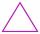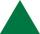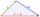# Triangle P2

Can triangle have two right angles?

Result

#### Solution:Leave us a comment of example and its solution (i.e. if it is still somewhat unclear...):

Showing 0 comments:Be the first to comment!#### To solve this verbal math problem are needed these knowledge from mathematics:

See also our right triangle calculator. See also our trigonometric triangle calculator.

## Next similar examples:

1. Area of RT 2Calculate the area of right triangle whose legs have a length 5.8 cm and 5.8 cm.
2. SimilarityAre two right triangles similar to each other if the first one has a acute angle 70° and second one has acute angle 20°?
3. Height 2Calculate the height of the equilateral triangle with side 38.
4. 3-bracket 3Two angles in a triangle are 90° and 60°. Has triangle at least two equal sides?
5. 3-bracket 2May be the smallest angle in the triangle greater than 40°?
6. QuizQAn isosceles triangle has two sides of length 7 km and 39 km. How long is a third side?
7. MidpointsTriangle whose sides are midpoints of sides of triangle ABC has a perimeter 45. How long is perimeter of triangle ABC?
8. TriangleDetermine if it is possible to construct a triangle with sides 28 31 34 by calculation.
9. MedianThe median of the triangle LMN is away from vertex N 84 cm. Calculate the length of the median, which start at N.
10. Write decimalsWrite in the decimal system the short and advanced form of these numbers: a) four thousand seventy-nine b) five hundred and one thousand six hundred and ten c) nine million twenty-six
11. Forest nurseryIn the forest nursery after winter, they found that 1/10 stems died out of them. For them, they land 193 new spruces. How many spruces are in the forest nursery?
12. ExpressionSolve for a specified variable: P=a+4b+3c, for a
13. Fraction to decimalWrite the fraction 3/22 as a decimal.
14. ZdeněkZdeněk picked up 15 l of water from a 100-liter full-water barrel. Write a fraction of what part of Zdeněk's water he picked.
15. In fractionsAn ant climbs 2/5 of the pole on the first hour and climbs 1/4 of the pole on the next hour. What part of the pole does the ant climb in two hours?
16. LineCan we construct a line segment, if we know: center
17. SimplifySimplify the following problem and express as a decimal: 5.68-[5-(2.69+5.65-3.89) /0.5]